Next: Optimising the cost function Up: Evaluating the cost function Previous: The likelihood term   Contents

### The terms originating from the hidden state sequence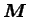The term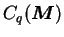is just a sum over the discrete distribution. It can be further simplified into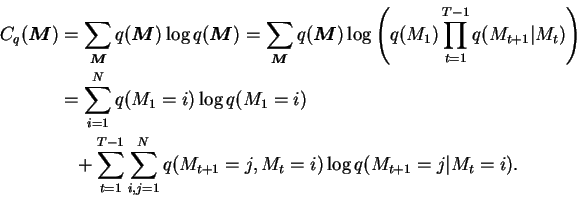(6.9)

The other term,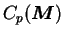can be split down to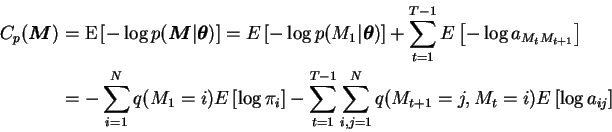(6.10)

where according to Equation (A.13),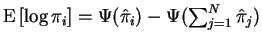and similarly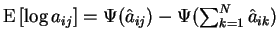.

The above equations give the value of the cost function for given approximating distribution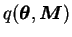. This value is important because it can be used to compare different models as shown in Section 3.3. Additionally it can be used to monitor whether the iterative optimisation procedure has converged.

Antti Honkela 2001-05-30